Courses

# Sample Test: Quantitative Aptitude- 1

## 34 Questions MCQ Test CAT Mock Test Series | Sample Test: Quantitative Aptitude- 1

Description
This mock test of Sample Test: Quantitative Aptitude- 1 for CAT helps you for every CAT entrance exam. This contains 34 Multiple Choice Questions for CAT Sample Test: Quantitative Aptitude- 1 (mcq) to study with solutions a complete question bank. The solved questions answers in this Sample Test: Quantitative Aptitude- 1 quiz give you a good mix of easy questions and tough questions. CAT students definitely take this Sample Test: Quantitative Aptitude- 1 exercise for a better result in the exam. You can find other Sample Test: Quantitative Aptitude- 1 extra questions, long questions & short questions for CAT on EduRev as well by searching above.
QUESTION: 1

### The question is followed by two statements, I and II. Answer each question using the following instructions: Mark (1) if the question can be answered by using statement I alone but not by using statement II alone. Mark (2) if the question can be answered by using statement II alone but not by using statement I alone. Mark (3) if the question can be answered by using both the statements together. Mark (4) if the question can be answered by using either of the statements. Is xy < 5? I. x < 3 andy < 3 II. 1/2 < x < 2/3 and y2< 16

Solution:

Statement I alone is not sufficient as xy may or may not be less than 5.
Statement II restricts x to 1/2 < x < 2/3 and y to the interval - 4 < y< 4 .

Thus, largest possible value of xy would be less than 8/3, which is less than 5.

Thus, Statement II is sufficient to answer.
Hence, option 2.

QUESTION: 2

### If logxy =100 and log4x = 5, then the value of y is:

Solution:

logx = 5 ⇒ x = 45 = 210
Substituting the value of x we get,
logxy = 100 ⇒ y = x100
y = (210)100 = 21000
Hence, option 3.

QUESTION: 3

### Krunal played 11 matches in Indian Cricket T-20 championship. Average runs scored by him in 11 matches was 16. His runs scored in nth match was 2 less than that of the (n - l)th match. Find the average runs scored by him in third and ninth match.

Solution:

Runs scored by him forms A.R with common difference = 2.
He played 11 matches.

∴  Runs scored by him in 6th match = Average runs scored in 11 matches = 1 6

For an A.R with n = 11 (1st term + 11th term)/2 = average Similarly,
(2nd term + 10th term) / 2 = average and so on...
∴ Average runs socred in the 3rd match and the 9th match = Average runs scored in 11 matches = 16
Hence, option 3.

QUESTION: 4

Find the number of roots of the equation 2x2 / (x - 1) - 4 = (6x - 4) / (x - 1), where x ≠ 1 ?

Solution:

On simplification, the given equation becomes,

2x2 - 10x + 8 = 0 So, x = 1 and x = 4 Since x ≠ 1,

x = 4 is the only root of the given equation.

Hence, option 2.

QUESTION: 5

How many scalene triangles are there for which the length of all the sides (in cm) are integers and the perimeter is 24 cm?

Solution:

Let a, b and c be the sides of each triangle satisfying the given conditions. The triangle is scalene.
Let a < b < c a + b + c = 24, where a, b and c are integers.
• a + b + c < 3c ⇒ (a + b + c)/3 < c i.e.,
longest side of a scalene triangle must be more than one-third of the perimeter.
•  a + b > c ⇒ a + b + c > c + c ⇒ (a + b + c > c + c ⇒ (a + b + c)/2 > c i.e.,

longest side o f a scalene triangle is less than half of the perimeter of the trianlge.

So, (a + b + c)/3 < c < (a + b + c)/2 ⇒ 8 < c < 12

∴ c is 9, 10 or 11.

If c is 9, a + b = 15

∴ (a, b) = (7, 8)

If c is 10, a + b = 14

∴ (a, b) = (5, 9) or (6, 8)

If c is 11, a + b = 13

∴  (a, ft) = (3,10), (4, 9), (5, 8) or (6, 7)

(a, b, c) has 7 possible values.

Hence, option 3.

QUESTION: 6

A boat takes 5 hours to row downstream along the entire length of the river. If the speed of the stream increases by 1 km/hr and the speed of the boat remains the same, the boat will take 40 hours to row upstream along the entire length of the river. If the speed of boat in still water is 5 km/hr, find the length of the river (in km).

Solution:

Let the speed of stream be x km/hr.

∴ Length of the river = (5 + x) x 5 = (5x + 25) km

Also, [5 - (x + 1)] x 40 = 5x + 25

Solving this we get, x = 3 km/hr

∴ Length of the river = (5 x 3 + 25) = 40 km

Hence Option 2

QUESTION: 7

In an A.P., if the product of 25 and 25th term is equal to the product of 29 and 29th term. Then which of the following term of that A.P. is definately zero?

Solution:

Let a be the first term and d be the common difference of the A.P.

∴ 25{a + 24d) = 29(a + 28d)

∴ 4 a + 212 d= 0

∴ a + 53 d = 0 Hence, 54th term of that A.P. is definately zero.
Hence, option 2.

QUESTION: 8

Two varieties of tea costing Rs. 280 per kg and Rs. 180 per kg are mixed in certain ratio to form varieties P and Q. Now, P and Q are mixed in the ratio 1 : 2 to form variety R which is sold for Rs. 288 per kg at 20% profit. If P costs Rs. 200 per kg, how much tea costing Rs. 180 is present in 100 kg of variety Q

Solution:

R is sold at Rs. 288 at 20% profit.
∴ CP of R = 288/1.2 = Rs. 240
Let the quantity of P and Q be x and 2x.

As CP of P = Rs. 200 we get,
240 = 200x + (2x x CP of Q) / (x + 2x)

∴ CP of Q = Rs. 260
Now,
260 = (280a + 180b) / a + b
where a and b are the varieties of tea costing Rs. 280 and Rs. 180 respectively.
Solving this we get, a : b = 4: 1

Tea costing Rs. 180 in 100 kg of variety Q = (1/5) x 100 = 20 kg

QUESTION: 9

A leap year starts on a Sunday. What is the probability that if a day is chosen from that year, it is a Tuesday?

Solution:

A leap year has 366 days, i.e., 52 weeks and 2 days.
Therefore the year will have 52 Tuesdays.

∴ Required probabilty = 52/366 = 26/183

Hence, option 3.

QUESTION: 10

In a farm, each cow eats twice as much grass as each sheep. The cost of grass for 20 cows and 80 sheeps for 40 days is Rs. 1800. Find the cost of grass in Rs. for 40 cows and 20 sheeps for 36 days.

Solution:

Explanation : 36[20x+40(2x)]..........(1)

40[80x + 20(2x)] = 1800

40[80x + 40x] = 1800

40[120x] = 1800

4800x = 1800

x = 1800/4800

x = 0.375

Put the values of x in eq(1)

36[20(0.375) + 40(2*0.375)]

= 36(7.5 + 30)

= 36 * 37.5

= 1350

QUESTION: 11

There are three positive integers. The sum of the squares of the any two of these equals the sum of the product of these two numbers and the squares of the third number. How many of the three numbers must be equal?

Solution:

Let the numbers be a, b, c.
Now,
a2 + b2 = ab + c2 , a2 + c2 = ac + b2, b2 + c2 = bc + a2
2(a2+b2+c2) = 2(ab + bc + ca)
∴ (a - b)2 + (b - c)2 + (c - a)2 = 0

This is possible only when a - b = b - c = c - a = 0
∴  a = b = c
Hence, all three numbers must be equal.
Hence, option 3.

QUESTION: 12

Forth and fifth terms of a series in geometric progression are 378 and 1134 respectively. What is the first term of the series?

Solution:

Let the first term and common ratio be a and r respectively.
Forth term = ar3 = 378

Similarly, Fifth term = ar4 =1134 Now,

r = ar4lar3 = 1134/378 = 3

∴ a =1134/81 = 14

Hence Option 2

QUESTION: 13

In the following figure, a rectangle is attached with a quarter circle. Perimeter of the complete figure is 60 cm2. What is the area of the figure in cm2?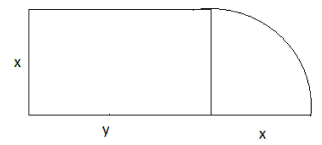Solution:Perimeter of the figure = 2x + 2y + 1/4(271 * x) = 60

Solving this, we get y = [ 240 - (8 + 2π)x]/8
Area of the figure = xy +1/4(πx2)
Substituting the value of y,
Area = x [240 - (8 + 2π)x]/8 + 1/4(πx2) = 30x - x2

QUESTION: 14

Each question is followed by two statements, I and II. Answer the question using the following instructions:

Mark the statement(s) that is needed to solve the question.

Find the LCM of x and y, where x and y are natural numbers.
I. Product of x and y is 899.
II. y is 29.

Solution:

From statement I: x andy are natural numbers, and their product is 899. So, only two cases are possible:

Case (i) One of them is 1 and other is 899.
Case (ii) One of them is 29 and other is 31.
In either case, the LCM of x and y = 899 So, statement I is sufficient to answer the question.

From statement II: Value of x is not known, so this statement alone is not sufficient to answer the question.

QUESTION: 15

A motorist used 15% of his fuel to cover 30% of his total journey. He covered another 40% of his total journey under similar conditions. For the rest of the journey conditions were different. Find the maximum percentage by which his fuel efficiency can drop, so that he can still cover the remaining journey without refill.Note: Fuel efficiency = distance covered per unit quantity of fuel.

Solution:

Let total fuel and total distance be 100 litres and 100 km respectively.

He covers 30 km in 15 litres.

Similarly, He covers 40 km in 20 litres.
Efficiency = Total distance/Total fuel consumption = 70/35 = 2 Remaining distance = 30 and remaining fuel = 65 litres.
Now, He will travel 30 km in 65 litres.

∴ Efficiency = 30/65 = 6/13
% change in efficiency = (2 - 6 / 13) / 2 x 100 = 76.92 %

QUESTION: 16

Four milkmen rented a pasture. A grazed 22 cows for 4 months, B grazed 12 cows for 5 months, C grazed 30 cows for 4 months and D grazed 21 cows for 3 months. If A’s share of rent is Rs. 880, then find the total rent (in Rs.) of the field.

Solution:

Let us calculate the total cow-months for each person.
A: 22 x 4 = 88 cow-months B: 12 x 5 = 60 cow-months C:30 x 4 = 1 2 0 cow-months D: 21 x 3 = 63 cow-months

∴Total = 331 cow-month Rent of 88 cow-months is Rs. 880

Hence, rent for 331 cow-months (i.e., entire field) = 880/88 x 331 = Rs. 3310

Hence Option 2

QUESTION: 17

The ratio of the cost price of cookie A to that of cookie B is 2 : 5. The ratio of profits made on selling the cookies is in the ratio 4 : 5. If the selling price of cookie B is Rs. 100, find the selling price of cookie A.

Solution:

Let the cost price for cookies A and B be 2x and 5x respectively and the profits for cookies A and B be 4y and 5y respectively.

Thus, for cookie B, SP = 5x + 5y = 100

∴ x + y = 20

For cookie A, SP = 2x + 4y But we do not know the value of x andy hence the selling price of cookie A cannot be determined.
Hence, option 4.

QUESTION: 18

A pole at point P on the boundary of a circular park subtends an angle of 30° at another point Q on the boundary. If the chord PQ subtends on angle of 60° at the center of the park, then find the ratio of height of the pole to the radius of the park.

Solution: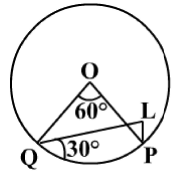Now,

PL = h and OQ = OP = a.
∴ PQ = a

∴ Δ PQL is a 30°-60°-90° triangle.
Now, PL = QP/√3

∴ PL : OP = PL : QP = 1 : V3
Hence, option 2.

QUESTION: 19

(4x2 + 2x) completely divides (8x3 + 8x2 + 2x). a is the quotient of the division. What is x if a is 3?

Solution:

(8x+ 8x2 + 2x) ÷ (4x2 + 2x) = (2x + 1)
a = 2x + 1
∴ 2x + 1 = 3 ⇒ x = 1
Hence Option 1

QUESTION: 20

There are two identical cubes (C1 and C2 ). C1 is perfectly cut into N identical small cubes. A sphere is inscribed in each of these cubes, such that it occupies maximum volume of the cube. Similarly, A sphere is also inscribed in C2 . The total volume occupied by the spheres in C1 is V 1 and that o f C2 is V2. Find V1 : V2.

Solution:

Let the side of a smaller cube is s and that of the bigger cube is b.
s3 x N =b3
Volume of Smaller sphere = 4 / 3πs3
Volume of bigger sphere, V2 = 4 / 3πb3
∴ V1 = N x 4 / 3πs3
V1 : V2 = N x 4 / 3πs3 : 4 / 3πb3 = 1 : 1
Hence, option 1.

QUESTION: 21

If a, b, c, d, e are positive real numbers, the minimum value of the product
(a + b + c + d + e) x [(1 / a) + (1 / b) + (1 / c) + (1 / d) + (1 / e)] is:

Solution:

We know that AM {a, b, c, d, e) ≥ HM (a, b, c, d, e)
∴ a + b + c + d + e / 5 ≥ 5 / [(1 / a) + (1 / b) + (1 / c) + (1 / d) + (1 / e)]
∴ (a + b + c + d + e) x [(1 / a) + (1 / b) + (1 / c) + (1 / d) + (1 / e)] ≥ 25

QUESTION: 22

A man standing at the bottom of a hill wants to reach the top, which is at a distance of 10√13 km from him. He starts climbing at an angle of 30°. After sometime he finds that he is 10 km above ground level and decides to climb the remaining distance at an angle of 60°. What is the height of the hill?

Solution: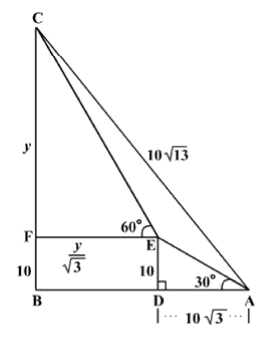DE = BF = 10 km
tan 60° = √3 = y / EF
∴ EF = y / √3 = DB
Let CF be y km.
tan 60° = √3 = y / EF
∴ EF = y / √3 = DB
AB = 10√3 + y / √3
BC = 10 +y
AC = 10√13 km
AB2+BC2 = AC2  ...(Pythagoras theorem)
(10√3 + y / √3)2 + (10 + y)2 = (10√13)2
Solving, y = 15 km

∴ Height = 10 + 15 = 25 km

Hence, option 3.

QUESTION: 23

A person has 9 beads of different colours. In how many unique ways can connect them to each other to form a necklace?

Solution:

To form a necklace, 9 beads can be arranged in (9 - 1)! = 8! ways We get a different arrangement if the necklace is turned upside down.
So, the actual number of different arrangements = 8!/2 = 20160 Answer: 20160

Hence Option 1

QUESTION: 24

Slant height of the cone is 25 cm. Diameter of the cone is 3/2 times the height of the cone. Find the volume of the cone (in cm3).

Solution:

Let x be the height of the cone.
∴ Diameter = 3x/2

So we get,
252 = x2 + (3x/4)2

∴ x = 20 cm

∴ Radius of the cone = (3 x 20)/4 = 15 cm

Volume of the cone = (1/3) x π x 152 x 20 = 1500π cm3
Hence, option 1.

QUESTION: 25

Sandeep, Shaunaq and Sahil started a business with investments of Rs. 8,000, Rs. 12,000 and Rs. 16,000 respectively. Shaunaq and Sahil left the business after x months. Out of the annual profit share, Sandeep got more than Shaunaq but less than Sahil. If x is an integer, find the the ratio of Sandeep, Shaunaq and Sahil’s shares.

Solution:

Ratio o f investement = 2 : 3 : 4 Marks Sandeep was in the business for whole year.

∴ Sandeep's share = 2 x 12 = 24
As Sandeep's share is multiple of 24, considering the options we get,
Ratio of shares = 24 : 21 : 28

Hence, option 2.

QUESTION: 26

Each question is followed by two statements, I and II. Answer each question 3 using the following instructions: Marks Mark (1) if the question can be answered by using statement I alone but not by using statement II alone.
Mark (2) if the question can be answered by using statement II alone but not by using statement I alone.
Mark (3) if the question can be answered by using either of the statements alone.
Mark (4) if the question can be answered by using both the statements together but not by either of the statements alone.
Mark (5) if the question cannot be answered by using any of the statements. Find the area of the triangle. (Enter the correct answer option.)

I. The measures of the sides of the triangle are in arithmetic progression.

II. The ratio of the sides is 2 : 3 : 4.

Solution:

The question cannot be answered using either of the statements alone. We need not combine the statements as statement II implies statement I.

Hence Option 5

QUESTION: 27

For which of the following functions f is f(x) = - f (1 +x) satisfies for all x?

Solution:

Let x = 4.
So we get,

f(4) = -f(5)
Now we will try the options.
Option 1: f(x) = 1 - x ⇒ f(4) = 1 - 4 = - 3 and - f(5) = -[1 - 5] = 4 As, f(4) ≠ - f(5)
Hence, option 1 is incorrect.
Option 2: f(x) = 1 - x2 ⇒ f(4) = 1 - (4)2 = 1 - 16 = -15 and -f(5) = -[1 - (5)2] = 24 As, f (4)  -f(5)
Hence, option 2 is incorrect.
Option 3: f(x) =x2(1 -x)2
f(4) = 1 - (4)2 = 1 - 4)2 = 16 x 9 and -f(5) = -(5)2 x [1 - (5)]2 = 25 x 16
∴ f(4) ≠ -f(5)
Hence, option 3 is incorrect.
Hence, option 4.

QUESTION: 28

A solid cylinder is immersed in a hemispherical bowl filled with water. What is the maximum volume of the liquid (in cubic units) that can be displaced, if the height of the cylinder is equal to the radius of the hemispherical bowl (given by r) and the radius of the cylinder is one third that of the bowl if cylinder is placed vertically?

Solution:

Consider the figure,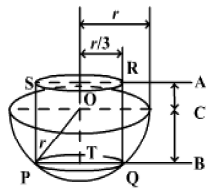The radius of hemispherical bowl = r Radius of cylinder below the surface of water = Radius of cylinder PQRS = r/3
Height of cylinder = r

Volume of the water displaced = Volume of the cylinder immersed.

Maximum height of the cylinder that can be immersed = AB - AC = BC

Using Pythagoras theorem in ΔPOT, we get,
∴ BC = 2√2r / 3
∴ Height of cylinder below the surface of water
= 2√2π / 3
∴ Volume of Liquid displaced = Volume of the cylinder immersed
= π x r2 / 9 x 2√2π / 3 = 2√2πr3 / 27
Hence, option 2.

QUESTION: 29

Consider the equation : ax2 + 12x - 27 = 0. If one of the roots of this equation is -3, then find the sum of the roots.

Solution:

Putting x = -3 in the equation, we get 9a - 36 - 27= 0

∴ a = 7

7x2 + 12x - 27 = 0
Thus, the sum of the roots of the quadratic equation = -12 / 7

QUESTION: 30

The average score of 12 students is 64. Three students scored the same as the average. The average score of four other students is 65 and the score of three other students is 62, 78 and 80 respectively. If the remaining two students had an equal score, then the score of each of the two is:

Solution:

Total score of 12 students = 64 x 12 = 768

Total score of 10 students = (3 x 64) + (4 x 65) + 62 + 78 + 80 = 672

Individual score of the remaining two students = (768 - 612)12 = 48

Hence Option 2

QUESTION: 31

A shopkeeper buys certain goods at 20% discount from the wholesaler. The shopkeeper then marks a price on the goods that would fetch him 50% profit. What percentage discount should he offer on this marked price so that he sells the goods at the marked price of the wholesaler (i.e., the price at which the wholesaler usually sells his goods) ?

Solution:

Let the marked price of the wholesaler be Rs. x.
After 20% discount, shopkeeper buys the goods at Rs. 0.8x To fetch 50% profit,
Shopkeeper marks the goods at 0.8x + 0.4x = Rs. 1.2x
To sell the goods at the marked price of wholesaler, shopkeeper should offer
∴ discount of 0.2x Discount percentage = (0.2x/1.2x) x 100 = 16.67%
Hence, option 2.

QUESTION: 32

In a class of 120 students, for every 2 students taking up Physics there are 4 students taking up Chemistry. Further for every student taking Physics there are 3 students taking up Maths as well as 1 student taking up Biology. Then the maximum number of students taking up all the four subjects will be:

Solution: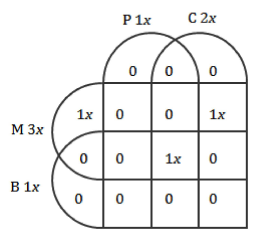Please refer to the Venn diagram. Here P stands for Physics, C stands for Chemistry, M stands for Maths and B stands for Biology.

We can write the given statements in form of ratios as following:
P C M B
2x 4x
1x 2x 3x 1x

∴The ratio of students taking up P:C:M:B will be 1:2:3:1.

Since we are supposed to maximize the number of students taking up all subjects, place lx the minimum of them in the intersection area of all four subjects. After placing the remaining students we get the values for different areas as given in the Venn diagram.

∴The total number of students will be 3x = 120

∴ x = 40
Hence, option 2.

QUESTION: 33

Everday, Murugan starts at 4:00 pm from his home to pick up his son from school. They reach house at 6:00 pm. One day, school was over at 4:00 pm and Murugan, not aware of this, started from home as usual. He met his son on the way and they reached home 20 minutes earlier than usual. If the speed of the car is 30 km/hr, find his son's speed (in km/hr).

Solution:

Murugan takes two hours to complete the journey in which he travels from home to school and back.

∴ Car takes 1 hour to travel from home to school and vice versa.
When son starts walking towards home, 20 minutes saved in whole journey i.e., 10 minutes journey from home to school and vice versa.

∴ Distance travelled by son = Distance travelled by car in 10 minutes.

As car takes 1 hour to reach from home to school of which 10 minutes distance is covered by son, car travels for 50 minutes from home to school.

∴ Son walks for 50 minutes.

Distance from school to car = 5 km

Speed of the boy = 6 km/hr

Hence, option 2.

QUESTION: 34

The difference between a two-digit number and the number obtained by interchanging the positions of its digits is 36. What is the difference between the two digits of that number?

Solution:

Let the ten's digit be x and unit's digit be y.

Then, (10x + y) - (10y + x) = 36

⇒ 9(x - y) = 36

⇒ x - y = 4